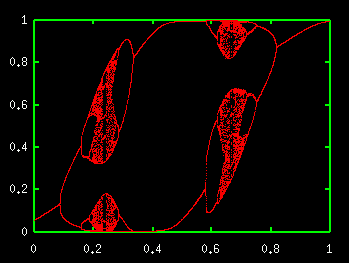# Bifurcation Diagram of Chaotic NeuronAs a single neuron model, we use

#### u(t+1)=0.7u(t)-0.9x(t)+a x(t)=1/[1+exp(-u(t)/0.06)]

where u(t) is membrane potential and x(t) is firing rate. This model is called chaos neuron. It yields chaos and the bifurcation phenomenon is observed like logistic map.

<< Bifurcation Diagram of Logistic Map

Introduction to Chaos and Nonlinear Dynamics